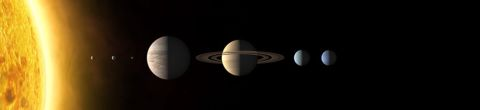# Classical MechanicsFall, 2011

Our exploration of the theoretical underpinnings of modern physics begins with classical mechanics, the mathematical physics worked out by Isaac Newton (1642--1727) and later by Joseph Lagrange (1736--1813) and William Rowan Hamilton (1805--1865). We will start with a discussion of the allowable laws of physics and then delve into Newtonian mechanics. We then study three formulations of classical mechanics respectively by Lagrange, Hamiltonian and Poisson. Throughout the lectures we will focus on the relation between symmetries and conservation laws. The last two lectures are devoted to electromagnetism and the application of the equations of classical mechanics to a particle in electromagnetic fields.  (Image credit: The International Astronomical Union/Martin Kornmesser)

## Lectures in this Course

1. ### State diagrams and the nature of physical laws

This first lecture is a general discussion of the nature of the laws of physics and in particular classical mechanics. The notions of configuration, reversibility, determinism, and conservation law are introduced for simple systems with a finite... [more]
2. ### Newton's law, phase space, momentum and energy

This lecture focuses on classical mechanics expressed using Newton's 2nd law. The notions of phase space, momentum and energy are introduced.
3. ### Lagrangian, least action, Euler-Lagrange equations

This lecture introduces Lagrange's formulation of classical mechanics. That formulation is formal and elegant; it is based on the Least Action Principle. The concepts introduced here are central to all modern physics. The lecture ends with angular... [more]
4. ### Symmetry and conservation laws

This lecture focuses on the relation between continuous symmetries of the Lagrangian and conserved quantities. Generalized coordinates and canonical conjugate momentum are introduced.
5. ### The Hamiltonian

This lecture starts with a thorough review of symmetries and conservation laws. Energy conservation is shown to be a consequence of time translation symmetry and the Hamiltonian is introduced.
6. ### Hamilton's equations

In this lecture many mechanical practical examples are worked out. Hamilton's equations are introduced: they represent one more way to do classical mechanics. The harmonic oscillator is revisited using Hamilton's equations.
7. ### Liouville s theorem

This lecture analyses the flow in phase space of multiple systems, and that flow is shown to be incompressible. Poisson brackets are introduced as yet another way to express classical mechanics formally.
8. ### Poisson brackets

Poisson brackets are another formal formulation of classical mechanics. They help make the connection between symmetries and conservation laws more explicit. The Poisson bracket of the x,y,z components of angular momentum are derived.
9. ### Electric and magnetic fields 1

This lecture introduces the static electric and magnetic fields, the associated Lagrangian and the Lorentz force. The vector potential, it's gauge field and gauge invariance are also introduced.
10. ### Electric and magnetic fields 2

This final lecture is a general review of all the concepts learned so far applied to a particle in electric and magnetic static fields.